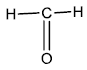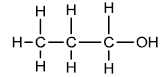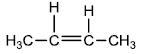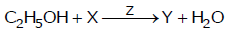Request a call back

# ICSE Class 10 Chemistry Previous Year Question Paper 2018

Chemistry is an essential subject in ICSE Class 10, as the fundamentals studied now will also be helpful in competitive exams like IIT-JEE and NEET. The ICSE Class 10 Chemistry syllabus includes concepts on Periodic tables, Chemical bonding, Study of acids, bases and salts, metallurgy, organic chemistry, and many more. Each of these concepts is very important for higher grades.

TopperLearning presents study materials for ICSE Class 10 Chemistry to help students score well in the examination. All our study materials are created by Experienced and qualified subject experts and give fundamental insight on how and what to prepare for the final exams.

ICSE Class 10 Test section is recommended for students as it consists of MCQs and SAQs types of questions, which will help boost the practice required for related exams.

All the content resources are created by experienced and qualified faculty members who have handled students with their studies and aided in clarifying their academic doubts through the ‘UnDoubt’ platform. Get access to these crucial student-centric resources through ICSE Class 10 Plans.

Question 1

(a) Choose the comet answer from the options given below:

(i) The salt solution which does not react with ammonium hydroxide is:

A. Calcium Nitrate

B. Zinc Nitrate

D. Copper Nitrate

(ii) The organic compound which undergoes substitution reaction is:

A. C2H2

B. C2H4

C. C10H18

D. C2H6

(iii) The electrolysis of acidified water is an example of:

A. Reduction

B. Oxidation

C. Redox reaction

D. Synthesis

(iv) The IUPAC name or dimethyl ether is:

A. Fumy methane

B. Methoxy methane

C. Methoxy ethane

D. Ethoxv ethane

(v) the catalyst used in the Contort Process is:

A. Copper

B. Iron

D. Manganese dioxide

(b) Give one word or a phrase for the hallowing statements:

(i) The energy released when an electron is added to a neutral gaseous isolated atom to from a negatively charged ion.

(ii) Process of formation of ions from molecules which are not in ionic state.

(iii) The tendency of an element to form chains of identical atoms.

(iv) The property by which certain hydrated salts, when left exposed to atmosphere, lose their water of crystallisation and crumble into powder.

(v) The process by which sulphide ore is concentrated.

(c) Write a balanced chemical equation for each of the following:

(i) Action of concentrated sulphuric acid on carbon.

(ii) Reaction of sodium hydroxide solution with iron (III) chloride solution.

(iii) Action of heat on aluminium hydroxide.

(iv) Reaction of zinc with potassium hydroxide solution.

(v) Action of dilute hydrochloric acid on magnesium sulphite.

(d)

(i) Given the IUPAC name for each of the following:

1.2.3.(ii) Write the structural formula of the isomers butane.

(e) State one relevant observation for each of the following:

(i) Lead nitrate solution is treated with sodium hydroxide solution drop wise till it is in excess.

(ii) At the anode, when molten lead bromide is electrolyzed using graphite electrodes.

(iii) Lead nitrate solution is mixed with dilute hydrochloric acid and heated.

(iv) Anhydrous calcium chloride is exposed to air for some time.

(v) Barium chloride solution is slowly added to sodium sulphate solution.

(f) Give a reason for each of the following:

(i) Ionic compounds have a high melting point.

(ii) Inert gases do not form ions.

(iii) Ionisation potential increases across a period, from left to right.

(iv) Alkali metals are good reducing agents.

(v) Conductivity of dilute hydrochloric acid is greater than that of acetic acid.

(g) Name the gas that is produced in each of the fallowing cases:

(i) Sulphur is oxidized by concentrated nitric acid.

(ii) Action of dilute hydrochloric acid on sodium sulphide.

(iii) Action of cold and & dilute nitric acid on copper.

(iv) At the anode during the electrolysis of acidified water.

(v) Reaction of ethanol and sodium.

(h) Fill up the blanks with the correct choice given in brackets.

(i) Ionic or electrovalent compounds do not conduct electricity in their ________ state fused/ solid)

(iii) Electrolysis of aqueous sodium chloride solution will form ________ at the cathode. (hydrogen gas / sodium metal)

(iv) Dry hydrogen chloride gas can be collected by ________ displacement of air. (downward / upward)

(v) The most common ore of iron is _________. (calamine / haematite)

(vi) The salt prepared by the method of direct combination is ________. (iron (II) chloride/  ron (III) chloride)

Question 2

(a)

(i) What do you understand by a lone pair of electrons?

(ii) Draw the electron dot diagram of Hydronium ion. (H=1; O=8)

(b) In Period 3 of the Periodic table, element B is placed to the left of element A. On the basis of this information, choose the correct word from the brackets to complete the following statements:

(i) The element B would have (lower /higher) metallic character than A.

(ii) The element A would probably have (lesser / higher) electron affinity than B.

(iii) The element A would have (greater /smaller) atomic size than B.

(c) Copy and complete the following table which refers to the conversion of ions to neutral particles.

 Conversion Ionic Equation Oxidation / Reduction Chloride ion to chlorine molecule (i) …………………… (ii) …………………… Lead (II) ion to lead (iii) …………………… (iv) ……………………

Question 3

(a)

(i) Write the balanced chemical equation to prepare ammonia gas in the laboratory by using an alkali.

(ii) State why concentrated sulphuric acid is not used for drying ammonia gas.

(iii) Why is ammonia gas not collected over water?

(b)

(i) Name the acid used for the preparation of hydrogen chloride gas in the laboratory. Why is this particular acid preferred to other acids?

(ii) Write the balanced chemical equation for the laboratory preparation of hydrogen chloride gas.

(c) For the preparation of hydrochloric acid in the laboratory:

(i) Why is direct absorption of hydrogen chloride gas in water not feasible?

(ii) What arrangement is done to dissolve hydrogen chloride gas in water?

(d) For the electro-refining of copper:

(i) What is the cathode made up of?

(ii) Write the reaction that takes place at the anode.

Question 4

(a) The percentage composition of a gas is:

Nitrogen 82.35% Hydrogen 17.64%

Find the empirical formula of the gas.[N = 14, H = 1]

(b) Aluminium carbide reacts with water according to the following equation.

Al4C3 + 12H2O → 4Al(OH)3 + 3CH4

(i) What mass of aluminium hydroxide is formed from 12g of aluminium carbide?

(ii) What volume of methane s.t.p. is obtained from 12g of aluminium carbide?

[Relative molecular weight of AlaC3 = 144; Al(OH)3 = 78]

(c)

(i) If 150 cc of gas A contains X molecules, how many molecules of gas B will be present in 75 cc of B?

The gases A and B are under the same conditions of temperature and pressure.

(ii) Name the law on which the above problem is based.

(d) Name the main component of the following alloys:

(i) Brass

(ii) Duralumin

Question 5

(a)  Complete the following table which relates to the homologous series of hydrocarbons.

 General formula IUPAC name of the homologous series Characteristic bond type IUPAC name of the first member of the series CnH2n- 2 (A) ________ (B) ________ (C) ________ CnH2n + 2 (D)________ (E)________ (F)________

(b)

(i) Name the most common ore of the metal aluminium from which the metal is extracted. Write the chemical formula of the ore.

(ii) Name the process by which impure ore of aluminium gets purified by using concentrated solution of an alkali.

(iii) Write the equation for the formation of aluminium at the cathode during the electrolysis of alumina.

Question 6

(a) A compound X (having vinegar like smell) when treated with ethanol in the

presence of the acid Z, given a compound Y which has a fruity smell.

The reaction is:(i) Identify Y and Z.

(ii) Write the structural formula of X.

(iii) Name the above reaction.

(b) Ethane burns in oxygen to from CO2 and H2O according to the equation:

2C2H6 + 7O2 → 4CO2 + 6H2O.

If 1250 cc of oxygen is burnt with 300 cc ethane.

Calculate:

(i) The volume of CO2 formed.

(ii) The volume of unused CO2.

(c) Three solutions P, Q and R have pH value of 3.5, 5.2 and 12.2 respectively.

Which one of these is a:

(i) Weak acid?

(ii) Strong alkali?

Question 7

(a) Given a chemical test to distinguish between the following pairs of chemicals:

(i) Lead nitrate solution and Zinc nitrate solution

(ii) Sodium chloride solution and Sodium nitrate solution

(b) Write a balanced equation for the preparation of each or the following salts:

(i) Copper sulphate from Copper carbonate

(ii) Zinc carbonate from Zinc sulphate

(c)

(i) What is the type of salt formed when the reactants are heated at a suitable temperature for the preparation of nitric acid?

(ii) State why for the preparation of nitric acid, the complete apparatus is made up of glass.

(d) Which property of sulphuric and is shown by the reaction of concentrated sulphuric acid with:

(i) Ethanol?

(ii) Carbon?

## Key Features of Study Materials for ICSE Class 10 Chemistry:

• According to the latest ICSE syllabus
• Prepared by subject matter experts